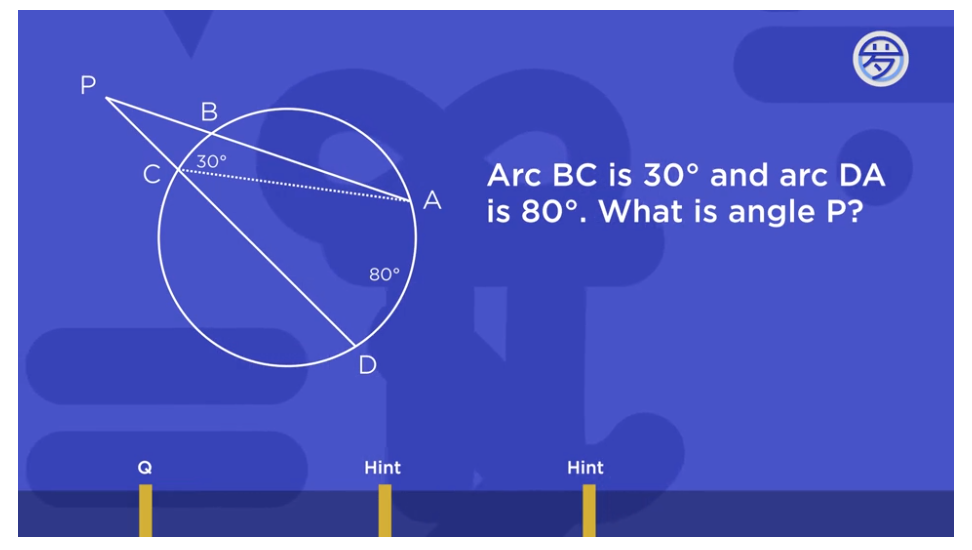# Why doesn't P just open up to the 80 degree angle as an inscribed angle? Would've made things a lot quicker, also doesn't it make sense?

• Why doesn't P just open up to the 80 degree angle as an inscribed angle? Would've made things a lot quicker, also doesn't it make sense?

• @The-Blade-Dancer You are right, using the Inscribed Angle Theorem would work a lot faster.That theorem tells us that we can take the difference of the two arcs that are inscribed by angle $$P,$$ and divide that by $$2$$ to get the measure of $$\angle P.$$$$\angle P = \frac{80^{\circ} - 30^{\circ}}{2} = \frac{50^{\circ}}{2} = \boxed{25^{\circ}}$$

You've finished the course already and are just reviewing, and so this theorem, which is proved in Day 10, isn't something that the kids know about when they're doing the Day 6 lesson. Maybe that's why Prof. Loh tried to use other methods to solve it!That's my guess, but I also know that Prof. Loh likes to use different solution methods to check his work, also.